# SEPIC/Ćuk converter sprouts second output

## Texas Instruments LM3488

Gheorghe Plasoianu

EDN

Many applications require positive and negative supply voltages, with only one voltage requiring tight regulation. This Design Idea describes a dual-output, hybrid SEPIC-Ćuk converter whose positive output voltage can be lesser or greater than the input voltage (Figure 1). The unregulated negative output is a mirrored replica of the positive output.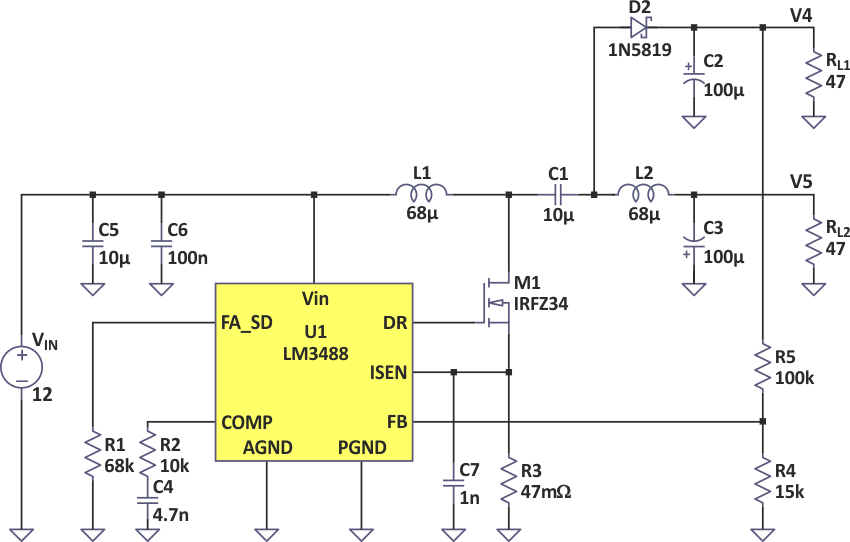Figure 1. Dual-output hybrid SEPIC-Ćuk converter.

To find out the output voltages we apply the principle of inductor volt-second balance and capacitor charge balance. To simplify the calculus, we neglect the voltage drop over the MOSFET and diode and we consider only continuous conduction mode.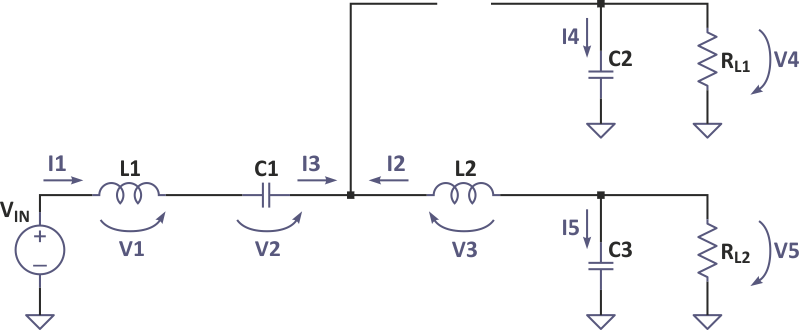Figure 2. Equivalent circuit in switch on-state.

When the MOSFET is on we have this equivalent network, shown in Figure 2, and the inductor voltages and capacitor currents for this interval, using small-ripple approximation, are: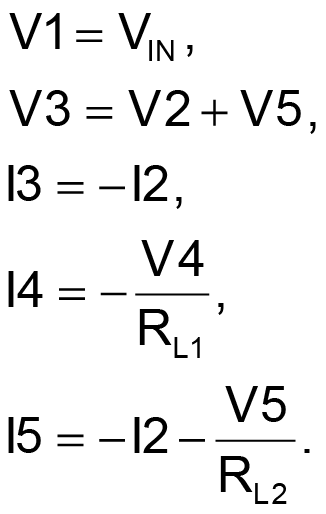When the MOSFET is off we have this equivalent network, shown in Figure 3, and the inductor voltages and capacitor currents for this interval are: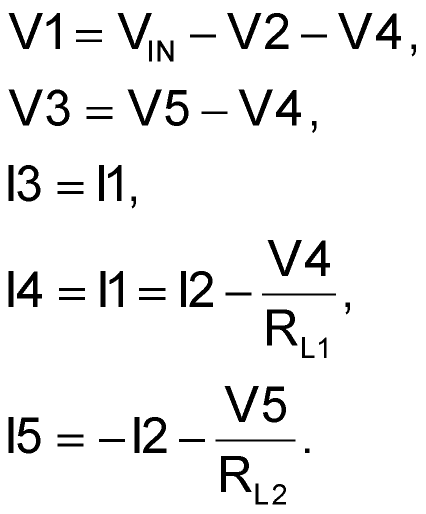Equating the average inductor voltages and capacitor currents over one switching period to zero, we get: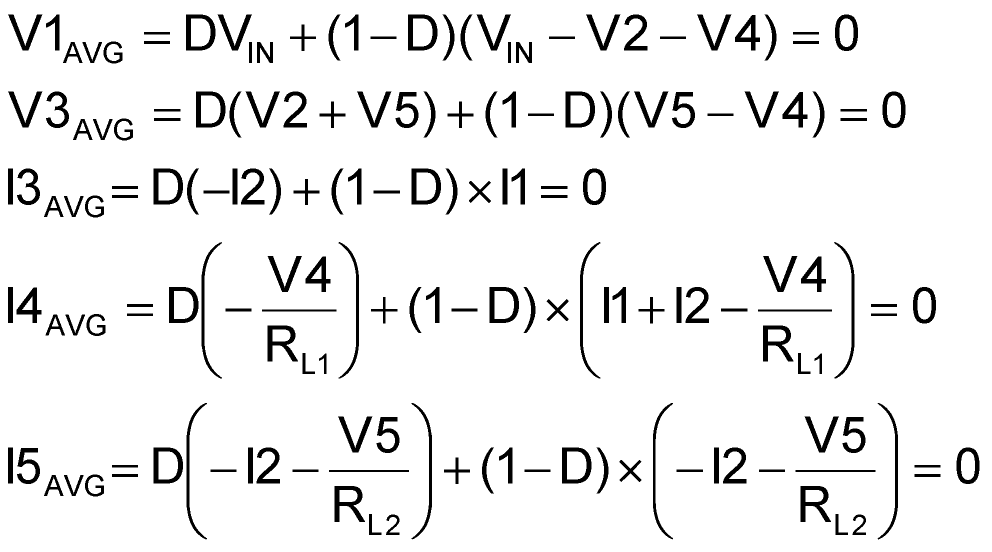where D is the duty cycle.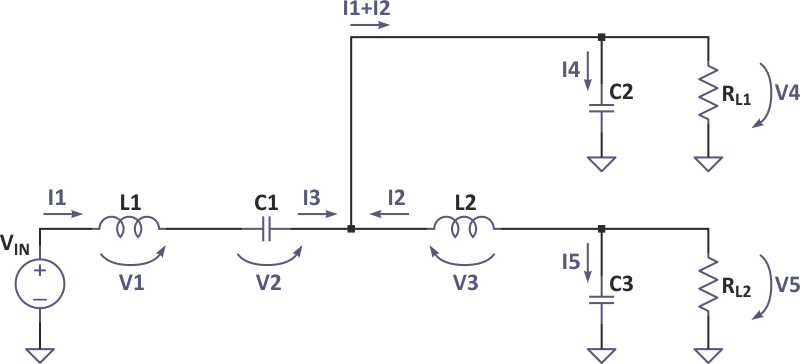Figure 3. Equivalent circuit in switch off-state.

Solving for V4 and V5: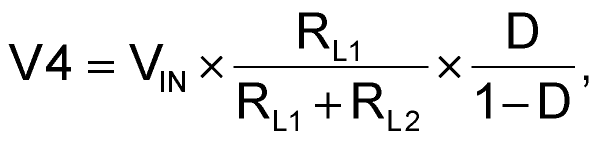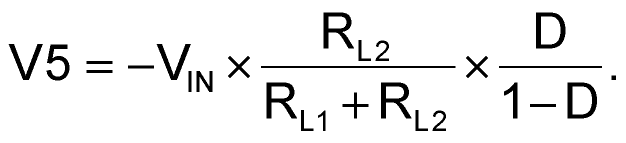In practice, due to the feedback, the positive output voltage, V4, is fixed.

Extracting the duty cycle from the V4 equation and inserting it into V5 results in: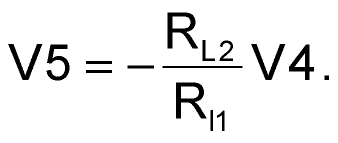Therefore, this topology is most suitable when the output currents do not differ much.

When the two loads are equal, then: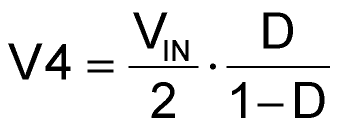and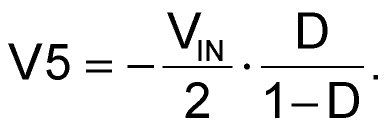A variation of the topology can supply a “floating” load is shown in Figure 4.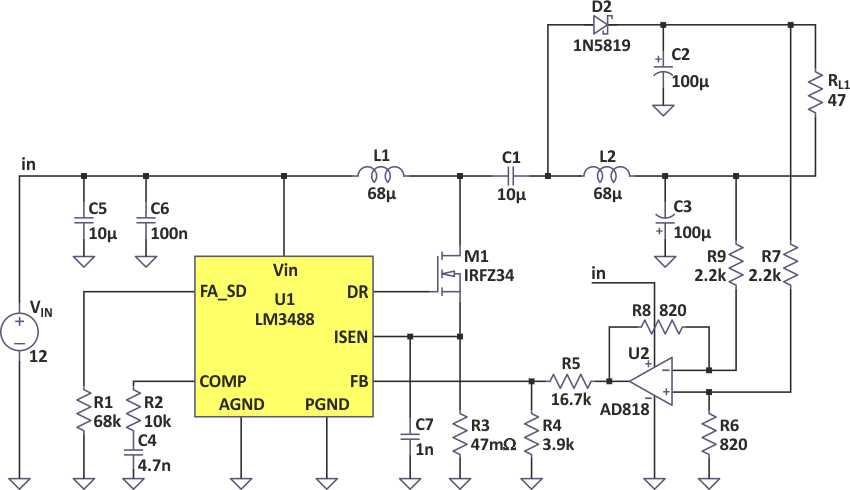Figure 4. Feedback modification for ungrounded load.

The op-amp converts the differential output voltage to single-ended for regulation. The differential output voltage is: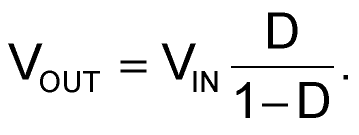For Figure 4: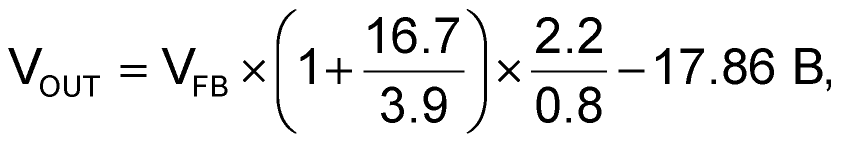where VFB of the LM3488 is 1.26 V.

I measured VOUT = 18 V for RL = 47 Ω, and 18.02 V with a 94 Ω load. That gives a load regulation of about 0.1 V/A, or 0.55% of VOUT.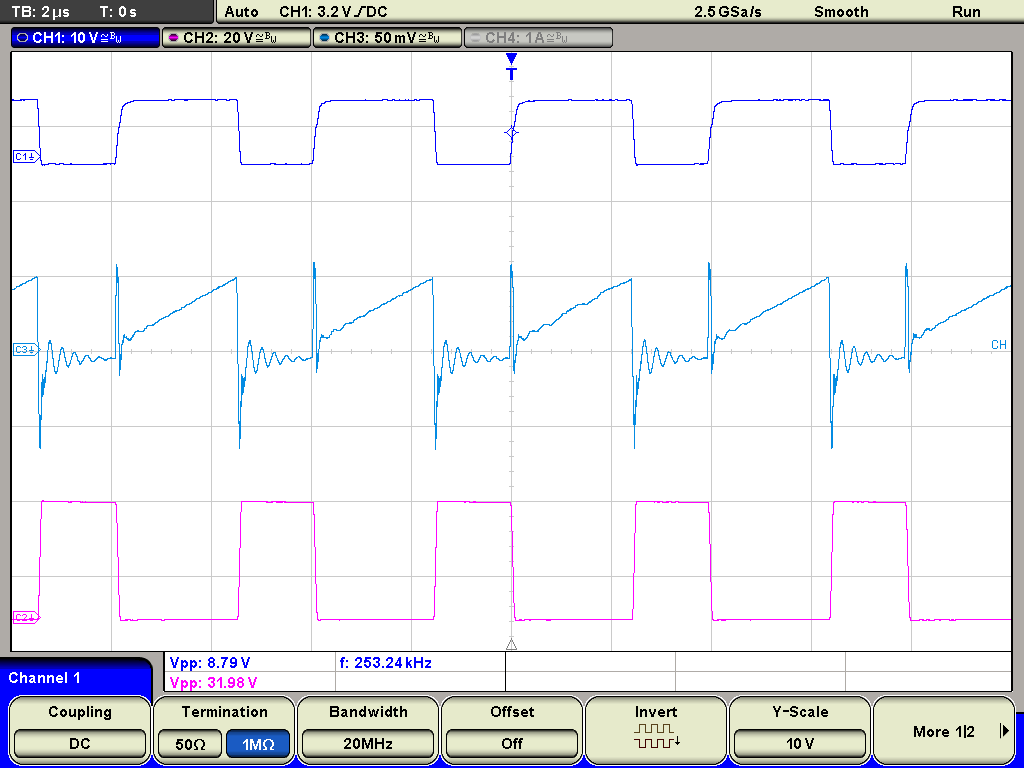Figure 5. MOSFET waveforms.

Figure 5 shows the gate, source, and drain voltages of the MOSFET. The duty cycle is about 60%.

dev.edn.com